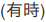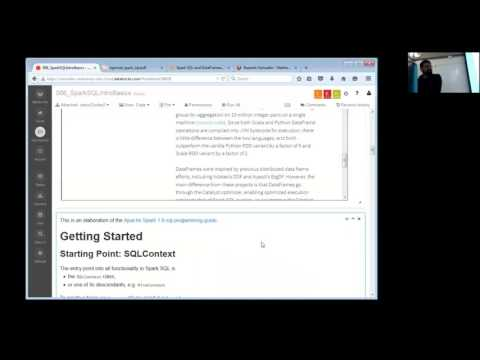// Databricks notebook source exported at Sat, 18 Jun 2016 08:58:54 UTC

# Scalable Data Science

### prepared by Raazesh Sainudiin and Sivanand Sivaram

The html source url of this databricks notebook and its recorded Uji:### Power Plant ML Pipeline Application - DataFrame Part

This is the Spark SQL parts of an end-to-end example of using a number of different machine learning algorithms to solve a supervised regression problem. This is a break-down of Power Plant ML Pipeline Application

This will be a recurring example in the sequel*

• Step 3: Explore Your Data
• Step 4: Visualize Your Data
• Step 5: Data Preparation
• Step 6: Data Modeling
• Step 7: Tuning and Evaluation
• Step 8: Deployment

We are trying to predict power output given a set of readings from various sensors in a gas-fired power generation plant. Power generation is a complex process, and understanding and predicting power output is an important element in managing a plant and its connection to the power grid.

• Given this business problem, we need to translate it to a Machine Learning task.
• The ML task is regression since the label (or target) we will be trying to predict is numeric.

Today, we will only cover Steps 1, 2, 3 and 4 above. You need introductions to linear algebra, stochastic gradient descent and decision trees before we can accomplish the applied ML task with some intuitive understanding. If you can’t wait for ML then check out Spark MLLib Programming Guide for comming attractions!

The example data is provided by UCI at UCI Machine Learning Repository Combined Cycle Power Plant Data Set

You can read the background on the UCI page, but in summary:

• we have collected a number of readings from sensors at a Gas Fired Power Plant (also called a Peaker Plant) and
• want to use those sensor readings to predict how much power the plant will generate in a couple weeks from now.
• Again, today we will just focus on Steps 1-4 above that pertain to DataFrames.

``````
//This allows easy embedding of publicly available information into any other notebook
//when viewing in git-book just ignore this block - you may have to manually chase the URL in frameIt("URL").
//Example usage:
// displayHTML(frameIt("https://en.wikipedia.org/wiki/Latent_Dirichlet_allocation#Topics_in_LDA",250))
def frameIt( u:String, h:Int ) : String = {
"""<iframe
src=""""+ u+""""
width="95%" height="""" + h + """"
sandbox>
<p>
<a href="http://spark.apache.org/docs/latest/index.html">
Fallback link for browsers that, unlikely, don't support frames
</a>
</p>
</iframe>"""
}
displayHTML(frameIt("https://en.wikipedia.org/wiki/Peaking_power_plant",300))

``````
``````
displayHTML(frameIt("https://archive.ics.uci.edu/ml/datasets/Combined+Cycle+Power+Plant",500))

``````
``````
require(sc.version.replace(".", "").toInt >= 140, "Spark 1.4.0+ is required to run this notebook. Please attach it to a Spark 1.4.0+ cluster.")

``````

##Step 1: Business Understanding The first step in any machine learning task is to understand the business need.

As described in the overview we are trying to predict power output given a set of readings from various sensors in a gas-fired power generation plant.

The problem is a regression problem since the label (or target) we are trying to predict is numeric

##Step 2: Load Your Data Now that we understand what we are trying to do, we need to load our data and describe it, explore it and verify it.

``````
display(dbutils.fs.ls("/databricks-datasets/power-plant/data")) // Ctrl+Enter

``````

Now let us load the data from the Tab-separated-variable or tsv text file into an `RDD[String]` using the familiar `textFile` method.

``````
val powerPlantRDD = sc.textFile("/databricks-datasets/power-plant/data/Sheet1.tsv") // Ctrl+Enter

``````
``````
powerPlantRDD.take(5).foreach(println) // Ctrl+Enter to print first 5 lines

``````
``````
// this reads the tsv file and turns it into a dataframe
.format("com.databricks.spark.csv") // use spark.csv package
.option("inferSchema", "true") // Automatically infer data types
.option("delimiter", "\t") // Specify the delimiter as Tab or '\t'

``````
``````
powerPlantDF.printSchema // print the schema of the DataFrame that was inferred

``````

### 2.1. Alternatively, load data via the upload GUI feature in databricks

Since the dataset is relatively small, we will use the upload feature in Databricks to upload the data as a table.

First download the Data Folder from UCI Machine Learning Repository Combined Cycle Power Plant Data Set

The file is a multi-tab Excel document so you will need to save each tab as a Text file export.

I prefer exporting as a Tab-Separated-Values (TSV) since it is more consistent than CSV.

Call each file Folds5x2_pp<Sheet 1..5>.tsv and save to your machine.

Go to the Databricks Menu > Tables > Create Table

Select Datasource as “File”

Upload ALL 5 files at once.

See screenshots below:

2.1.1. Create Table _________

When you import your data, name your table `power_plant`, specify all of the columns with the datatype `Double` and make sure you check the `First row is header` box.2.1.2. Review Schema ______

Your table schema and preview should look like this after you click `Create Table`:##Step 3: Explore Your Data Now that we understand what we are trying to do, we need to load our data and describe it, explore it and verify it.

#### Viewing as DataFrame

``````
display(powerPlantDF)

``````
``````
powerPlantDF.count() // count the number of rows in DF

``````

#### Viewing as Table via SQL

Let us look at what tables are already available, as follows:

``````
sqlContext.tables.show() // Ctrl+Enter to see available tables

``````

We need to register the DF as a temporary table before being able to access it via SQL.

``````
powerPlantDF.registerTempTable("power_plant_table") // Shift+Enter

``````
``````
sqlContext.tables.show() // Ctrl+Enter to see available tables

``````

Note that table names are in lower-case only!

You Try!

``````
sqlContext // put . after sqlContext and hit Tab to see what methods are available

``````
``````
//sqlContext.dropTempTable("power_plant_table") // uncomment and Ctrl+Enter if you want to remove the table!

``````

The following SQL statement simply selects all the columns (due to `*`) from `powerPlantTable`.

``````
%sql
-- Ctrl+Enter to query the rows via SQL
SELECT * FROM power_plant_table

``````

Note that the output of the above command is the same as `display(powerPlantDF)` we did earlier.

We can use the SQL `desc` command to describe the schema. This is the SQL equivalent of `powerPlantDF.printSchema` we saw earlier.

``````
%sql desc power_plant_table

``````

Schema Definition

Our schema definition from UCI appears below:

• AT = Atmospheric Temperature in C
• V = Exhaust Vaccum Speed
• AP = Atmospheric Pressure
• RH = Relative Humidity
• PE = Power Output

PE is our label or target. This is the value we are trying to predict given the measurements.

Reference UCI Machine Learning Repository Combined Cycle Power Plant Data Set

Let’s do some basic statistical analysis of all the columns.

We can use the describe function with no parameters to get some basic stats for each column like count, mean, max, min and standard deviation. More information can be found in the Spark API docs

``````
display(powerPlantDF.describe())

``````

To understand our data, we will look for correlations between features and the label. This can be important when choosing a model. E.g., if features and a label are linearly correlated, a linear model like Linear Regression can do well; if the relationship is very non-linear, more complex models such as Decision Trees can be better. We use the Databricks built in visualization to view each of our predictors in relation to the label column as a scatter plot to see the correlation between the predictors and the label.

``````
%sql select AT as Temperature, PE as Power from power_plant_table

``````

It looks like there is strong linear correlation between temperature and Power Output

``````
%sql select V as ExhaustVaccum, PE as Power from power_plant_table;

``````

The linear correlation is not as strong between Exhaust Vacuum Speed and Power Output but there is some semblance of a pattern.

``````
%sql select AP as Pressure, PE as Power from power_plant_table;

``````
``````
%sql select RH as Humidity, PE as Power from power_plant_table;

``````

…and atmospheric pressure and relative humidity seem to have little to no linear correlation.

These pairwise plots can also be done directly using `display` on `select`ed columns of the DataFrame `powerPlantDF`.

In general we will shy from SQL as much as possible. The illustations in `%sql` above are to mainly reassure those with a RDBMS background and SQL that their SQL expressibility can be directly used in Apache Spark and in databricks notebooks.

``````
display(powerPlantDF.select(\$"RH", \$"PE"))

``````

Furthermore, you can interactively start playing with `display` on the full DataFrame!

``````
display(powerPlantDF)

``````

We will do the following steps in the sequel.

• Step 5: Data Preparation
• Step 6: Data Modeling
• Step 7: Tuning and Evaluation
• Step 8: Deployment

Datasource References:

• Pinar Tüfekci, Prediction of full load electrical power output of a base load operated combined cycle power plant using machine learning methods, International Journal of Electrical Power & Energy Systems, Volume 60, September 2014, Pages 126-140, ISSN 0142-0615, Web Link
• Heysem Kaya, Pinar Tüfekci , Sadik Fikret Gürgen: Local and Global Learning Methods for Predicting Power of a Combined Gas & Steam Turbine, Proceedings of the International Conference on Emerging Trends in Computer and Electronics Engineering ICETCEE 2012, pp. 13-18 (Mar. 2012, Dubai) Web Link

Updated: